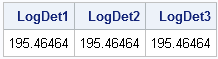The determinant of a matrix arises in many statistical computations, such as in estimating parameters that fit a distribution to multivariate data. For example, if you are using a log-likelihood function to fit a multivariate normal distribution, the formula for the log-likelihood involves the expression log(det(Σ)), where Σ is the covariance matrix for the population. Similar formulas appear for log-likelihood estimation of spatial models.

The determinant of a matrix is a high-degree polynomial, so it can be huge for even moderate-sized matrices. For example, the determinant of a Vandermonde matrix with consecutive integer elements increases super-factorially with the dimension of the matrix! For a 30 x 30 integer Vandermonde matrix, the determinant is too large to represent as a standard double-precision floating-point number.

But here's an important point: in many statistical applications, you don't care about the determinant. All you need is the logarithm of the determinant. That is a much, much, smaller number!

Here's a trick that you can use. To computing the logarithm of a determinant, do NOT try to compute the determinant itself. Instead, compute the log-determinant directly! For matrices with a large determinant, the computation of the log-determinant will usually succeed whereas the computation of the determinant might cause a numerical overflow error.

Here's how you can compute the log-determinant in the important case of a positive definite matrix, such as for a covariance matrix.

Let A be your matrix and let G = root(A) be the Cholesky root of the matrix A. Then the following equation is true:
log(det(A)) = 2*sum(log(vecdiag(G)))

Here's a proof:

• A = G`*G, by definition of the Cholesky root
• log(det(A)) = log(det(G`*G)) = log(det(G`)*det(G)) = 2*log(det(G))
• Since G is triangular, det(G) = prod(vecdiag(G))
• Therefore log(det(G))=sum(log(vecdiag(G)))
• Consequently, log(det(A)) = 2*sum(log(vecdiag(G)))

Here's how you can compute the log-determinant in the SAS/IML language:

```proc iml; start logdet(A); G = root(A); return( 2*sum(log(vecdiag(G))) ); finish;```

Let's use this formula on a 50x50 symmetric positive definite matrix. The following statements create a symmetric matrix with random uniform variates on the off-diagonal and the value 50 along the diagonal. This matrix is diagonally dominant, and therefore positive definite:

```n = 50; r = j(n*(n+1)/2, 1); /* allocate array for symmetric elements */ call randgen(r, "uniform"); /* fill r with U(0,1) */ A = sqrvech(r); /* A is symmetric */ diagIdx = do(1,n*n, n+1); A[diagIdx] = n; /* set diagonal elements */```

The determinant of A is about 7.75 x 1084. To test the log-determinant function, let's compute the log-determinant of A in three different ways: by calling the DET function, by computing eigenvalues, and by calling the logDet function:

```LogDet1 = log(det(A)); /* 1. DET function */ LogDet2 = log(prod(eigval(A))); /* 2. product of eigenvalues */ LogDet3 = logDet(A); /* 3. direct computation of log(det(A)) */   print LogDet1 LogDet2 LogDet3;```The first two methods compute the determinant, and are therefore subject to overflow when the determinant is extremely large. The logDet function, however, does not ever compute the determinant. It computes the log-determinant directly.

Sweet! This TRICK is a real TREAT!

ShareDistinguished Researcher in Computational Statistics

Rick Wicklin, PhD, is a distinguished researcher in computational statistics at SAS and is a principal developer of SAS/IML software. His areas of expertise include computational statistics, simulation, statistical graphics, and modern methods in statistical data analysis. Rick is author of the books Statistical Programming with SAS/IML Software and Simulating Data with SAS.

1.2.3.